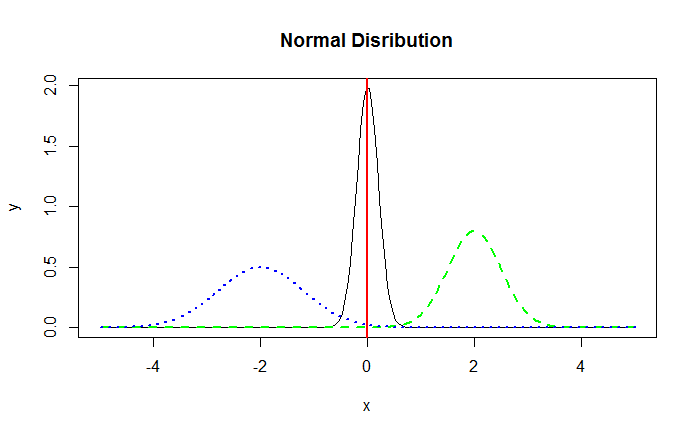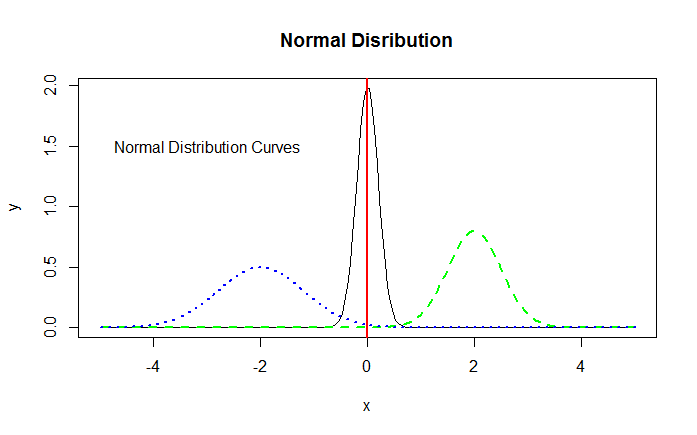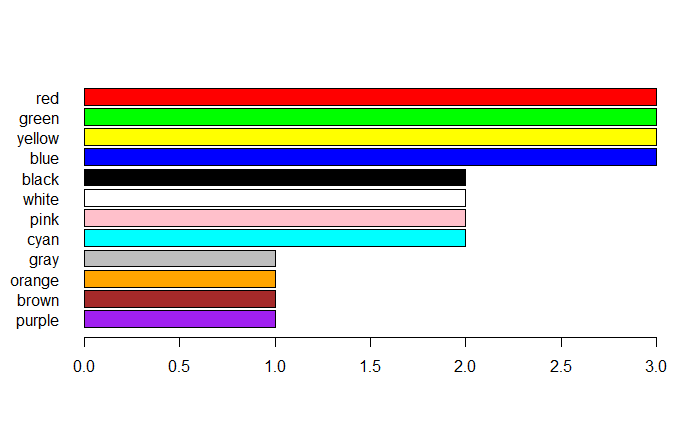# Adding Details and Features to R Plots

We've already seen how we can enhance the plots created in R by adding title, axis labels, legends, etc. Let's learn about a few more enhancements.

### Adding Vertical/Horizontal Lines to the Graph

You may want to add a vertical or a horizontal line to your graph at a certain axis point. This can be done using the abline() function.

> abline(v=0) # Draw a vertical line at x = 0.
> abline(h=0) # Draw a vertical line at y = 0.


In the below example, we add a vertical line at x=0 on our normal distribution plot.

> abline(v=0,col="red",lwd=2)


The resulting graph is displayed below:Normal Distribution - Vertical Line

### Adding Explanatory text to the Visualization

To make a data visualization useful for its users, it is important to add sufficient explanatory text to it. The function text() allows us to add text anywhere inside the plotting area.

The below code adds the text "Normal Distribution Curves" to our distributions graph.

> text(-3, 1.5, "Normal Distribution Curves")The text() function can be used to add text in many ways. Use the help ?text to learn more about the function.

### Using Colors Effectively

While designing data visualizations, it is important to use colors to easily distinguish between various data points. For example, if you have two lines on a plot, it's not a good idea to draw one as dark green and another as dark blue. It will be difficult to distinguish between the two lines. Iliinsky and Steele recommend a set of 12 colors, in descending order of desirability from the top of the plot to the bottom.

> # Iliinsky and Steele color name vector
> colors <- c("red", "green", "yellow", "blue", "black", "white", "pink", "cyan", "gray", "orange", "brown", "purple")
> data <- c(rep(3, 4), rep(2, 4),rep(1,4))
> barplot(rev(data), horiz = TRUE, col = rev(colors),names.arg = rev(colors), las = 1)


As you can see, we are using rep() in a function where we are creating our own sample data: rep() function replicates the values in x. For example, rep(3, 4) will repeat the value 3 4 times, i.e., 3 3 3 3. rev() function reverses an R object, including vector, array etc.Note that in the above graph, we use the argument las=1 to rotate the plot's axis labels. The las argument can have three possible values based on which the placement of the label will differ. With las equal to 0, the label will always be parallel to the axis (default); With las equal to 1, the label will be put horizontally. Value 2 will make it perpendicular to the axis and 3 will place it vertically.
las is actually an argument of the axis() function, that is, you can call the axis() function after the plot function to make changes to the axis. Apart from rotating the axis labels, it is capable of many things, such as changing the axis position, for example axis(3) will draw the x-axis above the plot area and axis(4) will draw the y-axis on the right side of the plot area. Note that if you want to edit the axis settings after plotting the graph using axis() function, it's a good idea to set the default axis to false (axes=FALSE) during the initial plot creation.